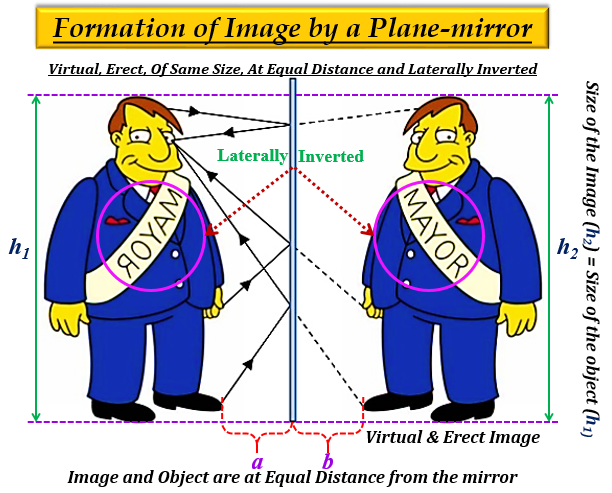# (a) List four characteristics of the images formed by plane mirrors.(b) A 5 cm tall object is placed at a distance of 20 cm from a concave mirror of focal length 30 cm. Use mirror formula to determine the position and size of the image formed.

(a) Characteristics of the image formed by a plane mirror:

1. The image formed by a plane mirror is virtual (behind the mirror) and erect (upright).

2. The size of the image formed by a plane mirror is the same as that of the size of the object.

3. The distance of the image formed by the plane mirror, is equal to the distance of the object from the mirror.

4The image formed by a plane mirror is laterally inverted, which means the right side of the object appears as the left side of its image and vice-versa (the left side of the object appears as the right side of its image).

Image is posted for reference only(b) Given: Concave Mirror

Object height, $h=+5cm$

Object distance, $u=-20cm$

Focal length, $f=-30cm$

To find:  Position, $v$ and size, $h'$ of the image formed.

Solution:

From the mirror formula we know that-

$\frac {1}{f}=\frac {1}{v}+\frac {1}{u}$

Substituting the required values, we get-

$\frac {1}{(-30)}=\frac {1}{v}+\frac {1}{(-20)}$

$-\frac {1}{30}=\frac {1}{v}-\frac {1}{20}$

$\frac {1}{20}-\frac {1}{30}=\frac {1}{v}$

$\frac {1}{v}=\frac {3-2}{60}$

$\frac {1}{v}=\frac {1}{60}$

$v=+60cm$

Thus, the position or distance of the image from the mirror is 60 cm, and the positive sign implies that the image forms behind the mirror (on the right).

Now,

From magnification formula we know that-

$m=\frac {-v}{u}=\frac {h'}{h}$

Substituting the required value, we get-

$\frac {-60}{-20}=\frac {h'}{5}$

$3=\frac {h'}{5}$

$h'=15$

Thus, the size or height of the image formed is 15cm (magnified).

Updated on: 10-Oct-2022

71 Views